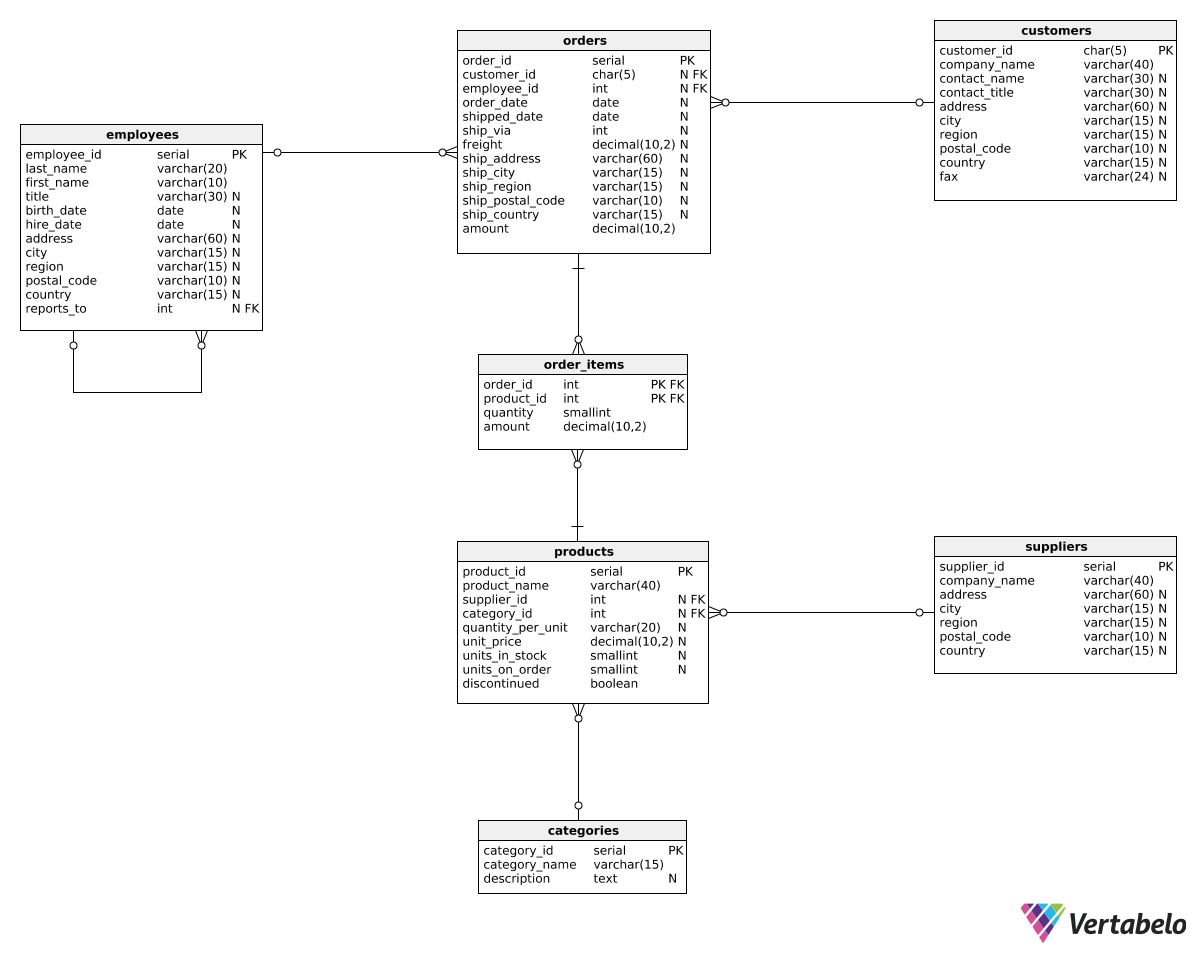Deals Of The Week - hours only!Up to 80% off on all courses and bundles.-Close
Introduction
Total revenue for multiple periods
Calculating deltas
Creating "revenue in quarters" reports
14. Creating columns with custom sums
Summary

## Instruction

Perfect! Now, let's say we want to create two columns which show different order sums based on the ship_country column. We can use the construction we've just learned, like so:

SELECT
SUM(CASE
WHEN ship_country IN ('USA', 'Canada', 'Mexico')
THEN amount
ELSE 0.0
END) AS sum_north_america,
SUM(CASE
WHEN ship_country NOT IN ('USA', 'Canada', 'Mexico')
THEN amount
ELSE 0.0
END) AS sum_elsewhere
FROM orders;


Above, we twice used the CASE WHEN construction from the previous exercise along with the SUM() function. For each row, CASE WHEN checks the value in the ship_country column. The order's amount is only added if the ship_country value matches the given condition. Otherwise, 0.0 is added. As a result, we'll get the total sum from orders shipped to the USA, Canada, and Mexico in the first column and the total sum from orders shipped to all other countries in the second column.

## Exercise

Show two columns:

1. sum_high_freight – The total amount generated by all orders with freight values above 100.0.
2. sum_low_freight – The total amount generated by all orders with freight values equal to or less than 100.0.

### Stuck? Here's a hint!

Calculate the first column as:

SUM(CASE
WHEN freight > 100
THEN amount
ELSE 0.0
END) AS sum_high_freight